# MEDseq: Mixtures of Exponential-Distance Models with Covariates

## Introduction

MEDseq is an R package which fits a range of models introduced by Murphy et al. (2019). These are finite mixtures of exponential-distance models for clustering longitudinal/categorical life-course sequence data. A family of parsimonious precision parameter constraints are accommodated. So too are sampling weights. Gating covariates can be supplied via formula interfaces. Visualisation of the results of such models is also facilitated.

The most important function in the MEDseq package is: MEDseq_fit, for fitting the models via the EM or CEM algorithms. MEDseq_control allows supplying additional arguments which govern, among other things, controls on the initialisation of the allocations for the EM/CEM algorithm and the various model selection options. MEDseq_compare is provided for conducting model selection between different results from using different covariate combinations &/or initialisation strategies, etc. MEDseq_stderr is provided for computing the standard errors of the coefficients for the covariates in the gating network.

A dedicated plotting function exists for visualising various aspects of the results, using new methods as well as some existing methods from the TraMineR package. Finally, the package also contains two data sets: biofam and mvad.

If you find bugs or want to suggest new features please visit the MEDseq GitHub issues page.

This vignette mostly aims to demonstrate the MEDseq models by reproducing the analysis of the MVAD data in the Murphy et al. (2019) paper. However, an additional example data set is also analysed.

### Installing MEDseq

MEDseq will run in Windows, Mac OS X or Linux. To install it you first need to install R. Installing Rstudio as a nice desktop environment for using R is also recommended.

Once in R you can type at the R command prompt:

install.packages('devtools')
devtools::install_github('Keefe-Murphy/MEDseq')

to install the latest development version of the package from the MEDseq GitHub page.

To instead install the latest stable official release of the package from CRAN go to R and type:

install.packages('MEDseq')

In either case, if you then type:

library(MEDseq)

it will load in all the MEDseq functions.

The GitHub version contains a few more features but some of these may not yet be fully tested, and occasionally this version might be liable to break when it is in the process of being updated.

Load the MVAD data. The data comes from a study by McVicar and Anyadike-Danes (2002) on transition from school to work. The data consist of static background characteristics (covariates and sampling weights) and a time series sequence of 72 monthly labour market activities for each of 712 individuals in a cohort survey. The individuals were followed up from July 1993 to June 1999. Type ?mvad for more information. We will drop the first sequence position (i.e. time point) as it (along with the covariates Grammar and Location) were used to define the sampling weights.

Note that the data set must have equal sequence lengths, and the intervals are assumed to be evenly spaced. The MVAD data meets these criteria. The TraMineR function seqdef is used to convert the data to the appropriate format.

data(mvad, package="MEDseq")
mvad$Location <- factor(apply(mvad[,5L:9L], 1L, function(x) which(x == "yes")), labels = colnames(mvad[,5L:9L])) mvad <- list(covariates = mvad[c(3L:4L,10L:14L,87L)], sequences = mvad[,15L:86L], weights = mvad[,2L]) mvad.cov <- mvad$covariates
mvad.seq      <- seqdef(mvad$sequences[,-1L], states = c("EM", "FE", "HE", "JL", "SC", "TR"), labels = c("Employment", "Further Education", "Higher Education", "Joblessness", "School", "Training")) The function MEDseq_control allows the algorithm used for model fitting, the method used to initialise the allocations (e.g. k-medoids or Ward’s hierarchical clustering), the criterion used to identify the optimal model (e.g. density-based silhouette (DBS), average silhouette width (ASW), BIC, etc.), and more to be specified. By default, the EM algorithm is employed, k-medoids is used to obtain starting values, and the (weighted) mean density-based silhouette criterion is used to choose the optimal model (if a range of models are fitted). In this vignette, we will mostly accept these defaults. The optimal model identified in the Murphy et al. (2019) paper has G=10 components, the UCN model type, sampling weights, and a subset of covariates identified using a stepwise variable selection procedure. The UCN model has a single precision parameter for each cluster. The gating covariates can be specified via a formula interface. The argument covars tells the formula where to look for the specified covariates. Thus, to fit such a model: mod1 <- MEDseq_fit(mvad.seq, G=10, modtype="UCN", weights=mvad$weights,
gating=~ fmpr + gcse5eq + livboth, covars=mvad.cov)

The names of the model types are CC, UC, CU, UU, CCN, UCN, CUN, and UUN. The first letter denotes whether the precision parameters are constrained (C) or unconstrained (U) across clusters, the second denotes the same across time periods, and the third letter (N) indicates the precision of a noise component. In this context, a noise component is one wherein the distribution of the sequences is uniform.

Typically, a range of G values and modtype settings are supplied to a single call to MEDseq_fit and chosen from using some criterion. Thus, for a given set of covariates, the model which is optimum in terms of the number of components and the precision parameter configuration can be identified.

To compare different runs using different sets of covariates, the function MEDseq_compare can be used. First, let’s fit models with all covariates included (except Grammar and Location) and no covariates included. Let’s try different numbers of components and different model types. Note that only the first model here includes a noise component.

# 9-component CUN model with no covariates.
# The CUN model has a precision parameter for each sequence position (i.e. time point),
# though each time point's precision is common across clusters.

mod2 <- MEDseq_fit(mvad.seq, G=9,  modtype="CUN", weights=mvad$weights, verbose=FALSE) # 11-component CC model with all coviarates. # The CC model has only a single precision parameter across all clusters and time points. mod3 <- MEDseq_fit(mvad.seq, G=11, modtype="CC", weights=mvad$weights,
gating=~. - Grammar - Location, covars=mvad.cov, verbose=FALSE)

Confirm that the first model is indeed optimal according to the (weighted) mean density-based silhouette. Examine the optimal model in greater detail. Observe how the UCN model type explicitly includes a noise component.

(comp <- MEDseq_compare(mod1, mod2, mod3, criterion="dbs"))
opt   <- comp$optimal summary(opt, classification = TRUE, parameters = FALSE, network = FALSE) ## --------------------------------------------------------------------- ## Comparison of Mixtures of Exponential-Distance Models with Covariates ## Data: mvad.seq ## Ranking Criterion: DBS ## Optimal Only: FALSE ## --------------------------------------------------------------------- ## ## rank MEDNames modelNames G df iters bic icl aic dbs ## 1 mod1 UCN 10 684 10 -86876.197 -86887.052 -83752.045 0.474 ## 2 mod2 CUN 9 647 49 -89379.589 -89388.574 -86424.433 0.42 ## 3 mod3 CC 11 852 5 -84801.298 -84816.481 -80909.81 0.238 ## asw loglik gating algo ## 0.359 -41192.022 ~fmpr + gcse5eq + livboth EM ## 0.37 -42565.217 None EM ## 0.329 -39602.905 ~male + catholic + funemp + gcse5eq + fmpr + livboth EM ## weights noise noise.gate equalPro ## TRUE TRUE TRUE ## TRUE TRUE FALSE ## TRUE FALSE ## ------------------------------------------------------ ## Mixture of Exponential-Distance Models with Covariates ## Data: mvad.seq ## ------------------------------------------------------ ## ## MEDseq (UCN), with 10 components ## Gating Network Covariates: ~fmpr + gcse5eq + livboth ## Noise Component: TRUE ## Noise Component Gating: TRUE ## ## log.likelihood N P V df iters DBS ASW BIC Algo ## -41192.02 712 71 6 684 10 0.47 0.36 -86876.2 EM ## ## Clustering table : ## 0 1 2 3 4 5 6 7 8 9 ## 16 79 39 46 138 155 65 30 57 87 Examine the estimated gating network coefficients. Note that standard errors can be computed by calling MEDseq_stderr on the opt object, to better inform the interpretations of the covariate effects. print(opt$gating)

Visualise the clusters uncovered by the optimal model. Note that seriation is applied for visual clarity. The legend indicates which colours correspond to which state categories.

plot(opt, type="clusters")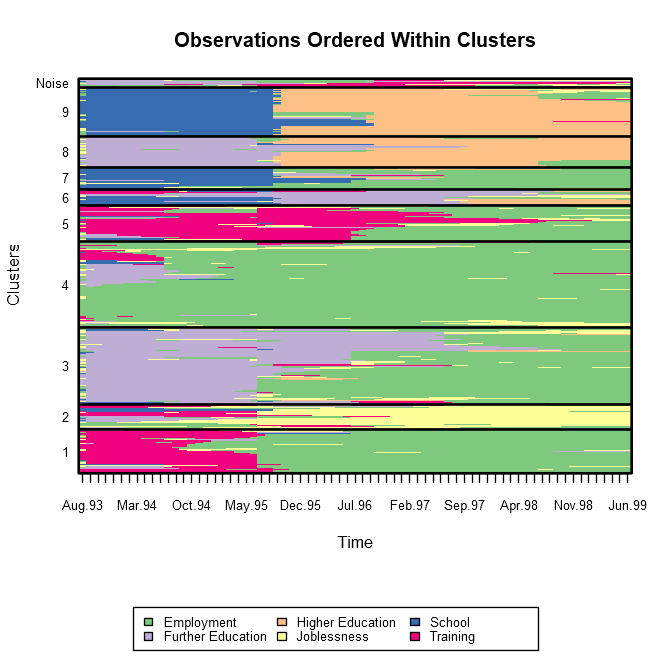Many other plotting options exist, some of which are adapted from the TraMineR package. Use the following code to examine the central sequence parameters. Note that the central sequence of the noise component is not shown as it doesn’t contribute to the likelihood.

plot(opt, type="mean")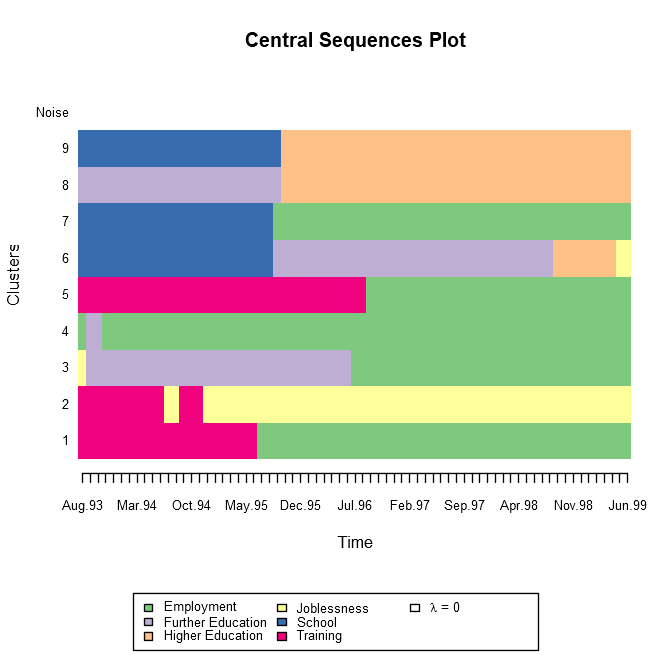Use the following code to see the observation-specific (weighted) density-based silhouette values (coloured by cluster). The (weighted) mean within each cluster is also shown. Note that the low average for the noise component is as expected; we do not expect this cluster to be internally coherent, rather it acts as a filter that allows the other clusters to be captured more clearly.

plot(opt, type="dbsvals")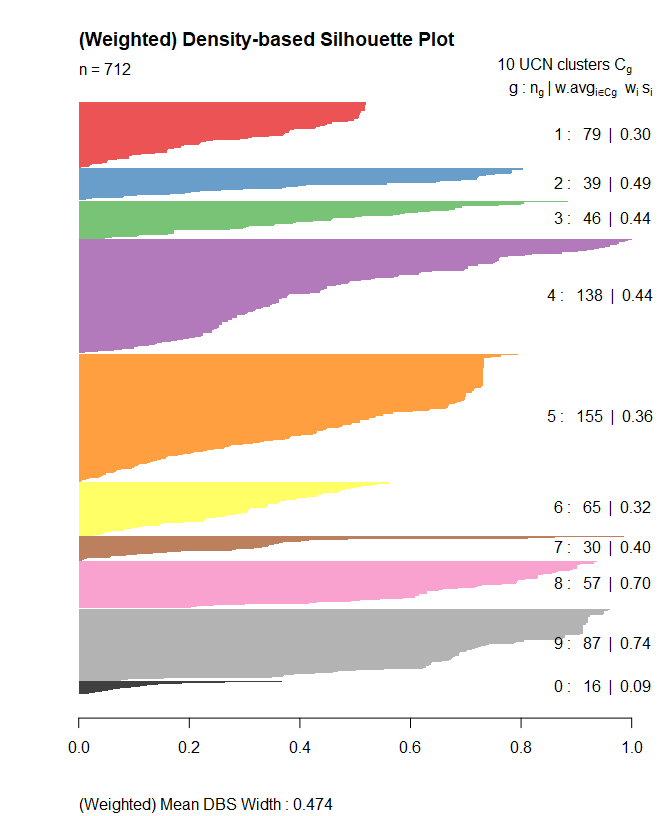Finally, we can quantify the type of observation characterising each cluster by computing the mean time spent in each state within each cluster. By specifying MAP=TRUE here, we are computing the mean time based on the hard MAP partition rather than the soft probabilistic assignments. By specifying norm=TRUE (which is the default), the mean times are normalised to sum to the sequence length within each cluster. The size of each cluster in terms of the number of observations assigned to it is also reported.

MEDseq_meantime(opt, MAP=TRUE, norm=TRUE)
##          Size         EM        FE        HE         JL         SC         TR
## Cluster1   79  1.7974684  0.000000 47.721519  2.3037975  0.5569620 18.6202532
## Cluster2   39  2.7179487  2.769231 37.435897  2.6923077 24.0000000  1.3846154
## Cluster3   46  4.0869565  0.000000  9.282609 44.5652174  2.8260870 10.2391304
## Cluster4  138 30.9782609  1.166667 33.644928  3.3188406  0.7318841  1.1594203
## Cluster5  155  2.9870968  0.000000 61.716129  3.6387097  0.4967742  2.1612903
## Cluster6   65  2.8153846  0.000000 28.230769  5.1076923  0.8923077 33.9538462
## Cluster7   30 33.3000000  7.366667  6.666667  4.1666667 16.1333333  3.3666667
## Cluster8   57 27.1929825 37.789474  4.456140  0.7719298  0.7894737  0.0000000
## Cluster9   87  0.5057471 38.034483  4.390805  1.3563218 26.4137931  0.2988506
## Noise      16 17.7500000  1.687500 14.187500 14.7500000  2.3125000 20.3125000

## Biofam Data

As a second example, let’s consider data on $$N=2000$$ 16 year-long family life state sequences built from the retrospective biographical survey carried out by the Swiss Household Panel (SHP) in 2002. Each of the $$v=8$$ states are defined from a combination of five basic states; namely, living with parents (Parent), left home (Left), married (Marr), having children (Child), and Divorced. The data is available in the MEDseq package as biofam. Type ?biofam for more information.

data(biofam, package="MEDseq")
biofam     <- list(covariates = biofam[2L:9L],
sequences = biofam[10L:25L] + 1L)
biofam.cov <- biofam$covariates[,colSums(is.na(biofam$covariates)) == 0]
biofam.seq <- seqdef(biofam\$sequences,
states = c("P", "L", "M", "L+M",
"C", "L+C", "L+M+C", "D"),
labels = c("Parent", "Left", "Married",
"Left+Marr", "Child", "Left+Child",
"Left+Marr+Child", "Divorced"))

While the data set contains weights, they are not appropriate for use; biofam is merely a subsample of the original data so the weights are not properly adapted. Thus, we will fit a model without supplying the weights argument. Secondly, the data set also contain some baseline covariates. As many of them contain missing values, let’s only consider the birthwt variable, which gives the birth year of each subject.

In the previous example, the model by default assumed that covariates also influenced the mixing proportion of the noise component. We can override this by specifying noise.gate=FALSE via MEDseq_control. Thus, the noise component’s mixing proportion will be constant (though estimated) across all observed sequences and covariate patterns.

# The UUN model includes a noise component.
# Otherwise, the model has a precision parameter for each time point in each cluster.

bio <- MEDseq_fit(biofam.seq, G=10, modtype="UUN",
gating=~ birthyr, covars=biofam.cov,
control=MEDseq_control(noise.gate=FALSE))

Such a model was identified as optimal according to the weighted DBS criterion following the same steps as the analysis of the MVAD data in the Murphy et al. (2019) paper. Print the details of the model to the screen by typing print(bio):

## Call:    MEDseq_fit(seqs = biofam.seq, G = 10, modtype = "UUN", gating = ~birthyr,
##     covars = biofam.cov, control = MEDseq_control(noise.gate = FALSE))
##
## Best Model: UUN, with 10 components and no weights (incl. gating network covariates)
## DBS = 0.498 | ASW = 0.392 | BIC = -48455.116 | ICL = -48584.227 | AIC = -46741.24
## Gating: ~birthyr

As before, let’s look at the clusters uncovered by the model. This time, seriate="both" means to order the clusters and the observations within clusters for visual clarity.

plot(bio, type="clusters", seriate="both")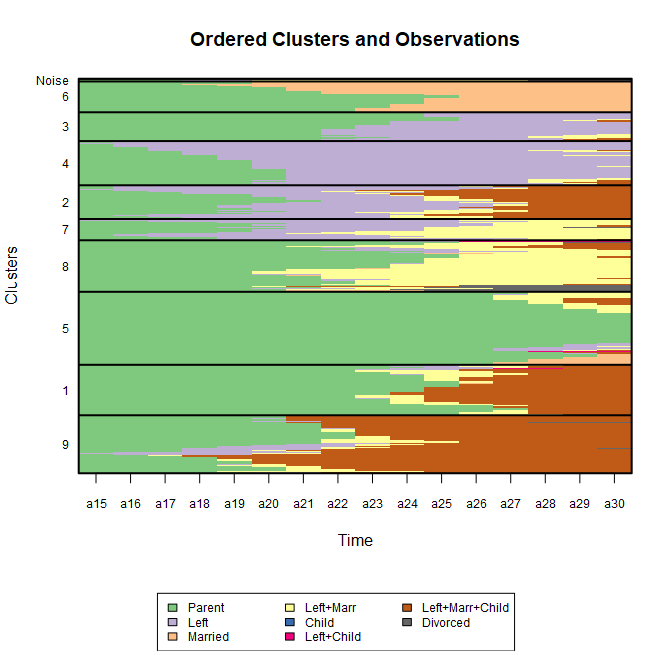Use the following code to examine the precision parameters. Note how we have a full $$16\times G$$ matrix of precision parameters, one for each time point in each cluster. Typically, we would not supply the argument log.scale=TRUE. However, in this case there are many quite large precision parameter values which skew the colour scale. Indeed, some are even infinite! Infinite precision under UU or UUN models implies that all values for that time point are identical within the given cluster.

plot(bio, type="precision", log.scale=TRUE)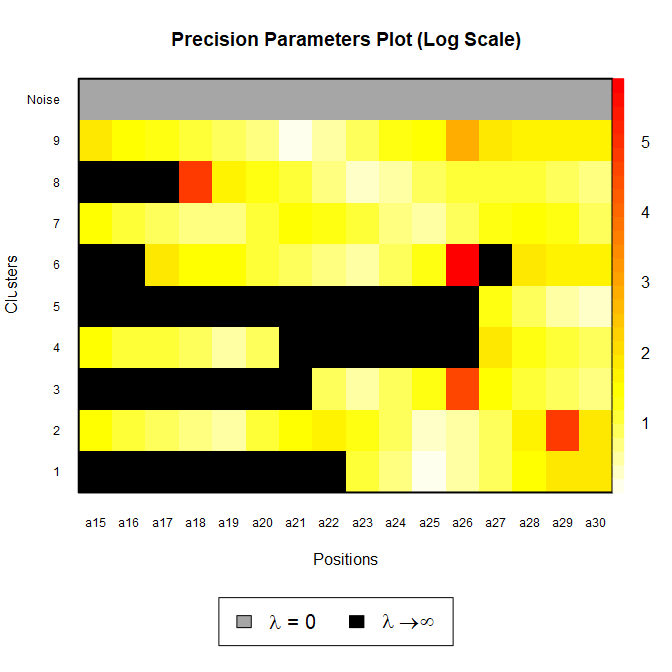This time, rather than showing the weighted mean DBS values, let’s look at observation-specific (unweighted) average silhouette-width values. Note that this relies instead on the MAP partition rather than the soft cluster assignment probabilities.

plot(bio, type="aswvals")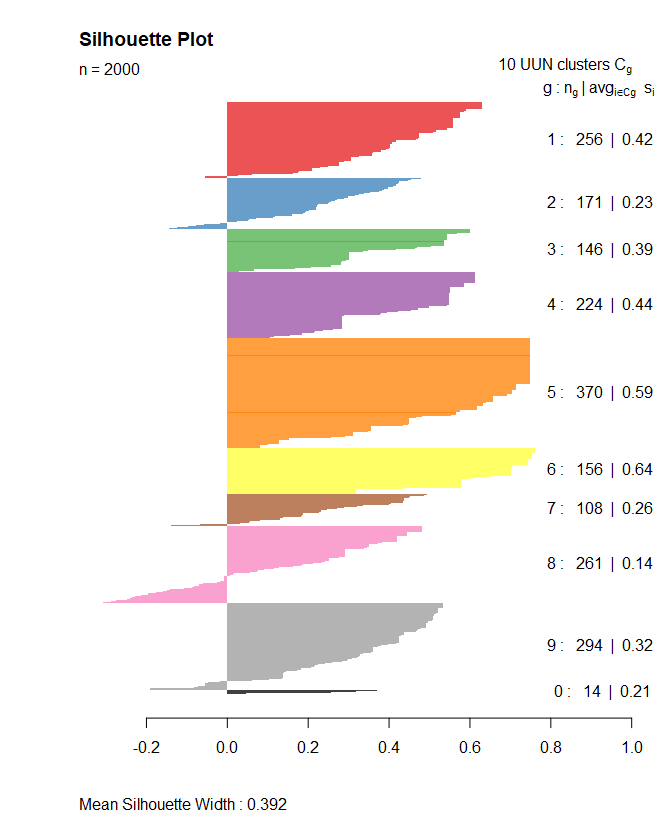As stated above, some plot types are adapted from TraMineR. Let’s first look at a plot of the transversal Shannon entropies for the whole data set.

seqHtplot(biofam.seq)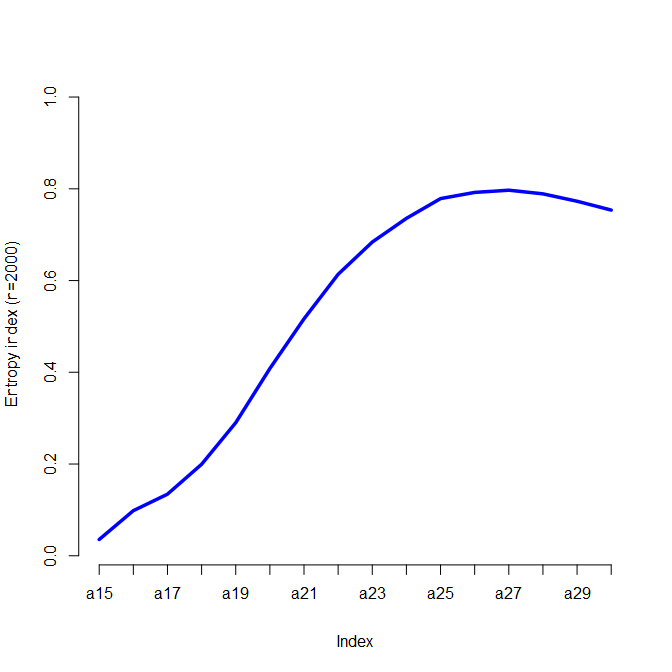Now let’s use the plot function from MEDseq to examine the transversal entropies within each cluster defined by the MAP partition. Here we can see for instance that subjects assigned to Cluster 9, corresponding to those individuals who left the parental home to marry relatively early and had a child on average just one year later, do indeed exhibit greater variability. Conversely, a postponement of the transition to adulthood is evident for subjects in Cluster 5.

plot(bio, type="Ht")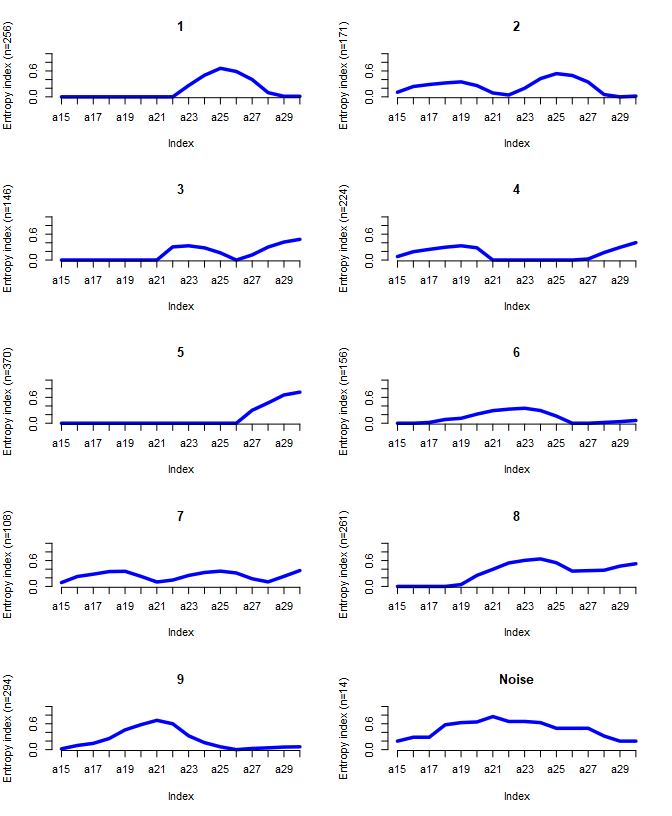Other plot types adapted from TraMineR can be produced using type="d" (state distribution plots), type="f" (state frequency plots), type="i" (selected sequence index plots), and type="I" (whole set index plots). Each of these plots are shown on a per-cluster basis. Clustering uncertainties, the gating network, and model selection criteria can also be visualised.